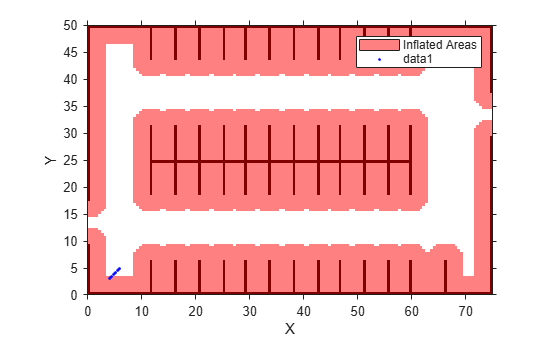# checkFree

Check vehicle costmap for collision-free poses or points

## Syntax

``free = checkFree(costmap,vehiclePoses)``
``free = checkFree(costmap,xyPoints)``
``freeMat = checkFree(costmap)``

## Description

The `checkFree` function checks whether vehicle poses or points are free from obstacles on the vehicle costmap. Path planning algorithms use `checkFree` to check whether candidate vehicle poses along a path are navigable.

To simplify the collision check for a vehicle pose, `vehicleCostmap` inflates obstacles according to the vehicle's `InflationRadius`, as specified by the `CollisionChecker` property of the costmap. The collision checker calculates the inflation radius by enclosing the vehicle in a set of overlapping circles of radius R, where the centers of these circles lie along the longitudinal axis of the vehicle. The inflation radius is the minimum R needed to fully enclose the vehicle in these circles.

A vehicle pose is collision-free when the following conditions apply:

• None of the vehicle's circle centers lie on an inflated grid cell.

• The cost value of each containing a circle center is less than the `FreeThreshold` of the costmap.

For more details, see the algorithm on the `vehicleCostmap` reference page.

example

````free = checkFree(costmap,vehiclePoses)` checks whether the vehicle poses are free from collision with obstacles on the costmap.```
````free = checkFree(costmap,xyPoints)` checks whether (x, y) points in `xyPoints` are free from collision with obstacles on the costmap.```
````freeMat = checkFree(costmap)` returns a logical matrix that indicates whether each cell of the costmap is free.```

## Examples

collapse all

Load a costmap from a parking lot.

```data = load('parkingLotCostmap.mat'); parkMap = data.parkingLotCostmap; plot(parkMap)```

Create vehicle poses following a straight-line path. `x` and `y` are the (x,y) coordinates of the rear axle of the vehicle. `theta` is the angle of the rear axle with respect to the x-axis. Note that the dimensions of the vehicle are stored in the `CollisionChecker.VehicleDimensions` property of the costmap, and that there is an offset between the rear axle of the vehicle and its center.

```x = 4:0.25:6; y = 3:0.25:5; theta = repmat(45,size(x)); vehiclePoses = [x',y',theta']; hold on plot(x,y,'b.') hold off```The first few (x,y) coordinates of the rear axle are within the inflated area. However, this does not imply a collision because the center of the vehicle may be outside the inflated area. Check if the poses are collision-free.

`free = checkFree(parkMap,vehiclePoses)`
```free = 9x1 logical array 1 1 1 1 1 1 1 1 1 ```

All values of `free` are 1 (`true`), so all poses are collision-free. The center of the vehicle does not enter the inflated area at any pose.

## Input Arguments

collapse all

Costmap, specified as a `vehicleCostmap` object.

Vehicle poses, specified as an m-by-3 matrix of [x, y, Θ] vectors. m is the number of poses.

x and y specify the location of the vehicle in world units, such as meters. This location is the center of the rear axle of the vehicle.

Θ specifies the orientation angle of the vehicle in degrees with respect to the x-axis. Θ is positive in the clockwise direction.

Example: `[3.4 2.6 0]` specifies a vehicle with the center of the rear axle at (3.4, 2.6) and an orientation angle of 0 degrees.

Points, specified as an M-by-2 real-valued matrix that represents the (x, y) coordinates of M points.

Example: `[3.4 2.6]` specifies a single point at (3.4, 2.6)

Example: `[3 2;3 3;4 7]` specifies three points: (3, 2), (3, 3), and (4, 7)

## Output Arguments

collapse all

Vehicle pose or point is free, returned as an M-by-1 logical vector. An element of `free` is `1` (`true`) when the corresponding vehicle pose in `vehiclePoses` or point in `xyPoints` is collision-free.

Costmap cell is free, returned as a logical matrix of the same size as the costmap grid. This size is specified by the `MapSize` property of the `costmap`. An element of `freeMat` is `1` (`true`) when the corresponding cell in `costmap` is unoccupied and the cost value of the cell is below the `FreeThreshold` of the costmap.

## Tips

• If you specify a small value of `InflationRadius` that does not completely enclose the vehicle, then `checkFree` might report occupied poses as collision-free. To avoid this situation, the default value of `InflationRadius` completely encloses the vehicle.

## Version History

Introduced in R2018a